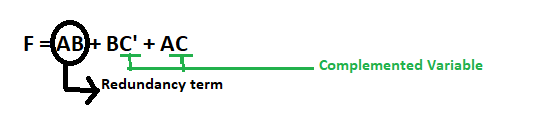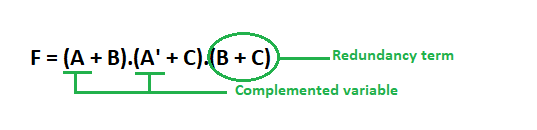# Consensus Theorem in Digital Logic

• Difficulty Level : Easy
• Last Updated : 13 Dec, 2019

Redundancy theorem is used as a Boolean algebra trick in Digital Electronics. It is also known as Consensus Theorem:

`  AB + A'C + BC = AB + A'C`

The consensus or resolvent of the terms AB and A’C is BC. It is the conjunction of all the unique literals of the terms, excluding the literal that appears unnegated in one term and negated in the other.

The conjunctive dual of this equation is:

`(A+B).(A'+C).(B+C) = (A+B).(A'+C)`

In the second line, we omit the third product term BC.Here, the term BC is known as Redundant term. In this way we use this theorem to simply the Boolean Algebra. Conditions for applying Redundancy theorem are:

1. Three variables must present in the expression.Here A, B and C are used as variables.
2. Each variables is repeated twice.
3. One variable must present in complemented form.

After applying this theorem we can only take those terms which contains the complemented variable.

Proof – We can also prove it like this:

```Y = AB + A'C + BC
Y = AB + A'C + BC.1
Y = AB + A'C + BC.(A + A')
Y = AB + A'C + ABC + A'BC
Y = AB(1 + C) + A'C(1 + B)
Y = AB + A'C             ```

Example-1.

`F = AB + BC' + AC`

Here, we have three variables A, B and C and all are repeated twice. The variable C is present in complemented form. So, all the conditions are satisfied for applying this theorem.After applying Redundancy theorem we can write only the terms containing complemented variables (i.e, C) and omit the Redundancy term i.e., AB.

` .'. F = BC' + AC`

Example-2.

`F = (A + B).(A' + C).(B + C)`

Three variables are present and all are repeated twice. The variable A is present in complemented form.Thus, all the three conditions of this theorem is satisfied.After applying Redundancy theorem we can write only the terms containing complemented variables (i.e, A) and omit the Redundancy term i.e., (B + C).

`.'. F = (A + B).(A' + C)`

Consider the following equation:

`Y = AB + A'C + BC`

The third product term BC is a redundant consensus term. If A switches from 1 to 0 while B=1 and C=1, Y remains 1. During the transition of signal A in logic gates, both the first and second term may be 0 momentarily. The third term prevents a glitch since its value of 1 in this case is not affected by the transition of signal A.

Thus. it is important to remove Logic Redundancy because it causes unnecessary network complexity and raises the cost of implementation.

So, in this way we can minimize a Boolean expression to solve it.

My Personal Notes arrow_drop_up
Recommended Articles
Page :